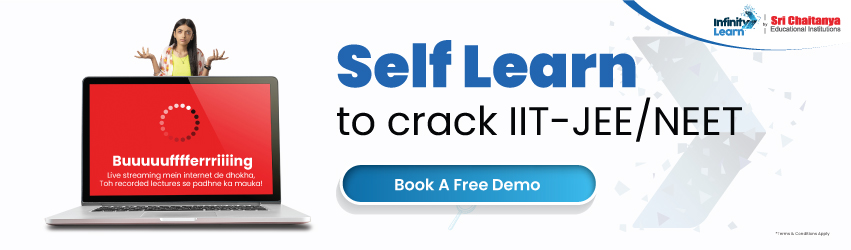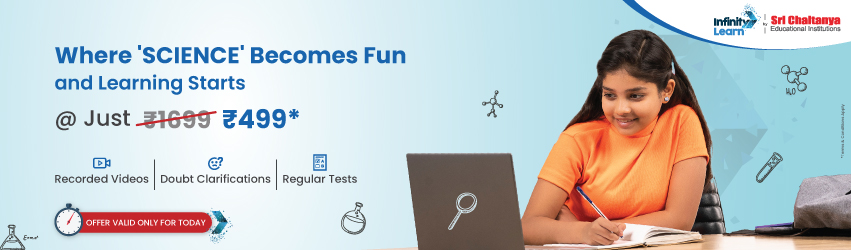NCERT Solutions for Class 11 Maths Chapter 3 Trigonometric Functions Miscellaneous Exercise

# NCERT Solutions for Class 11 Maths Chapter 3 Trigonometric Functions Miscellaneous Exerciseinfinitylearn surge self learn
infinitylearn starter package

All miscellaneous exercise questions are answered in NCERT Solutions for Class 11 Maths Chapter 3 miscellaneous exercise Trigonometric Functions. The principals studied in Maths chapter 11 are the foundation for NCERT Solutions for Class 11 miscellaneous exercise. This practice is crucial for both the CBSE Term II exam and competitive exams. To do well on your exam, download these NCERT Solutions and practice them offline.

Need FREE NCERT/CBSE/IIT-JEE/NEET Study Material?

NCERT Solutions for Class 11 Maths Chapter 3 Trigonometric Functions miscellaneous exercise

Using the NCERT solutions for miscellaneous exercise Class 11 Maths is the best option for the CBSE students when they are preparing for their exams. This chapter includes many exercises. One of them are the Exercise miscellaneous exercise whose solutions are available in pdf format on this page. You can also download these solutions online from our website or app. Infinity Learn subject matter experts have designed solution from the exercise with the utmost care and by keeping in mind all the guidelines by CBSE. Class 11 Students which have prepared with all the exercise given in this chapter can easily score well in the exams. By using these solutions, students came to know about the pattern of questions that are asked in the exam from this chapter and also know about the marks weightage of the chapter. So they can prepare well.

Along with this exercise, there are many number of exercises included in this chapter which contain innumerable questions as well. All these solutions are designed by subject matter experts as mentioned earlier. Hence all of these are considered as superior quality and students must refer to these solutions. To score well in the exam all these solution must be practised well and all other questions must be solved which are given next to it. Do not delay any more. Download the NCERT solutions for Class 11 Maths Chapter 3 miscellaneous exercise from Infinity Learn website now for better exam preparation. If you have the Infinity Learn app on your phone, you should download the same through the app as well. You can use these solutions online and offline as well

1. What will I Learn in this chapter of class 11 maths chapter 3?

Ans. Students will Learn about Degree of measure, Angles, Radian measure, Relations between Radian and Real numbers, Relation between Degree and Radian, Trigonometric Functions, Sign of Trigonometric Functions, Domain and Range of Trigonometric Functions, Trigonometric Functions of Sum and Difference of Two Angles and Trigonometric Equations. All this help to Learn how to find the radian measure of degree measures, how to find the degree measures of a radian measure, how to find the ratio of the radii of a circle, how to find trigonometric functions in quadrants and many more calculations.

1. Are NCERT Solutions of this exercise important from the exam point of view?

Ans. Yes, it is important for the students not to skip any exercise of this chapter from the NCERT textbook as all these exercises present in NCERT book of Class 11 Maths are crucial for exams as well as for good marks. So, students must frequently practice all the questions present in NCERT Solutions for miscellaneous exercise to make higher grades. These solutions are very easy to understand and is designed in simple language.

1. Is 11 Standard Maths easy?

Ans. Students frequently find the Maths of Class 11 very difficult. This is due to the big difference in the syllabus of Class 10 and Class 11. The concepts involved are new for them, and students fail to adapt this sudden change. So that’s why for scoring good marks, you must practice all the NCERT Solutions for Class 11 Maths well and do frequent revisions.

Join Infinity Learn Regular Class Programme!